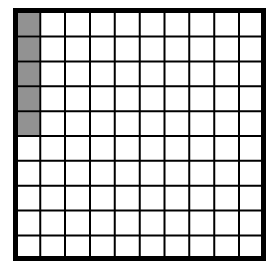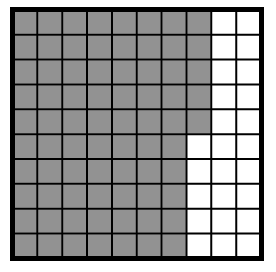### Home > ACC6 > Chapter 3 Unit 3 > Lesson CC1: 3.2.4 > Problem3-131

3-131.

The following representations have been drawn to represent portions of a 100% block. Write each of the portions in at least two different forms.

1.• How many blocks are filled in? How could you represent this in writing?

Since $5$ blocks out of $100$ are filled in, it can be expressed in two ways.

$\frac{5}{100}\text{ or } 0.05$

1.Use part (a) as a guide, because this problem is very similar.

How many blocks are filled in? What portion of the whole does this represent?